# IB Math Analysis & Approaches Questionbank-Topic: SL 2.3 The graph of a function SL Paper 1

## Question

Let $$f(x) = 3\sin \left( {\frac{\pi }{2}x} \right)$$, for $$0 \leqslant x \leqslant 4$$.

(i)     Write down the amplitude of $$f$$.

(ii)     Find the period of $$f$$.


a.

On the following grid sketch the graph of $$f$$.b.

## Markscheme

(i)     3     A1     N1

(ii)     valid attempt to find the period     (M1)

eg$$\,\,\,\,\,$$$$\frac{{2\pi }}{b},{\text{ }}\frac{{2\pi }}{{\frac{\pi }{2}}}$$

period $$= 4$$     A1     N2

[3 marks]

a.A1A1A1A1     N4

[4 marks]

b.

## Examiners report

Almost all candidates correctly stated the amplitude but then had difficulty finding the correct period. Few students faced problems in sketching the graph of the given function, even if they had found the wrong period, thus indicating a lack of understanding of the term ‘period’ in part a(ii). Most sketches were good although care should be taken to observe the given domain and to draw a neat curve.

a.

Almost all candidates correctly stated the amplitude but then had difficulty finding the correct period. Few students faced problems in sketching the graph of the given function, even if they had found the wrong period, thus indicating a lack of understanding of the term ‘period’ in part a(ii). Most sketches were good although care should be taken to observe the given domain and to draw a neat curve.

b.

## Question

The following diagram shows the graph of a function $$f$$, with domain $$– 2 \leqslant x \leqslant 4$$.The points $$( – 2,{\text{ }}0)$$ and $$(4,{\text{ }}7)$$ lie on the graph of $$f$$.

Write down the range of $$f$$.


a.

Write down $$f(2)$$;


b.i.

Write down $${f^{ – 1}}(2)$$.


b.ii.

On the grid, sketch the graph of $${f^{ – 1}}$$.


c.

## Markscheme

correct range (do not accept $$0 \leqslant x \leqslant 7$$)     A1     N1

eg$$\,\,\,\,\,$$$$[0,{\text{ }}7],{\text{ }}0 \leqslant y \leqslant 7$$

[1 mark]

a.

$$f(2) = 3$$     A1     N1

[1 mark]

b.i.

$${f^{ – 1}}(2) = 0$$     A1     N1

[1 mark]

b.ii.A1A1A1     N3

Notes:     Award A1 for both end points within circles,

A1 for images of $$(2,{\text{ }}3)$$ and $$(0,{\text{ }}2)$$ within circles,

A1 for approximately correct reflection in $$y = x$$, concave up then concave down shape (do not accept line segments).

[3 marks]

c.

## Question

Consider a function f (x) , for −2 ≤ x ≤ 2 . The following diagram shows the graph of f.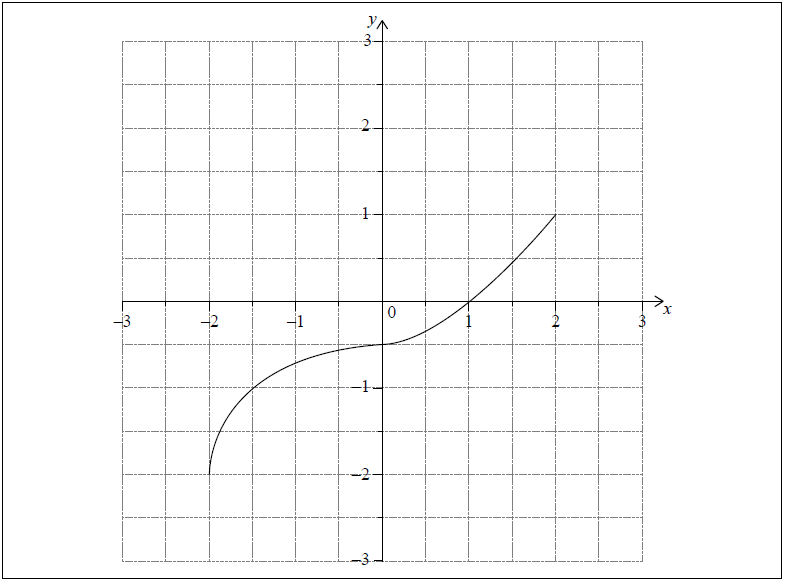Write down the value of f (0).


a.i.

Write down the value of f −1 (1).


a.ii.

Write down the range of f −1.


b.

On the grid above, sketch the graph of f −1.


c.

## Markscheme

$$f\left( 0 \right) = – \frac{1}{2}$$     A1 N1

[1 mark]

a.i.

f −1 (1) = 2     A1 N1

[1 mark]

a.ii.

−2 ≤ y ≤ 2, y∈ [−2, 2]  (accept −2 ≤ x ≤ 2)     A1 N1

[1 mark]

b.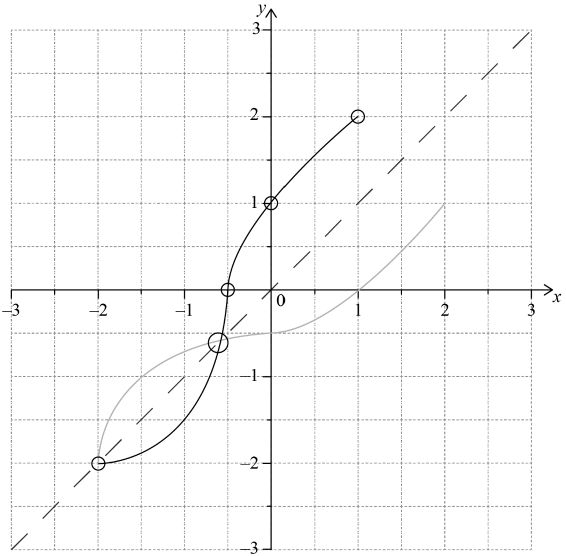A1A1A1A1  N4

Note: Award A1 for evidence of approximately correct reflection in y = x with correct curvature.

(y = x does not need to be explicitly seen)

Only if this mark is awarded, award marks as follows:

A1 for both correct invariant points in circles,

A1 for the three other points in circles,

A1 for correct domain.

[4 marks]

c.

## Question

The following diagram shows the graph of a function $$f$$, for −4 ≤ x ≤ 2.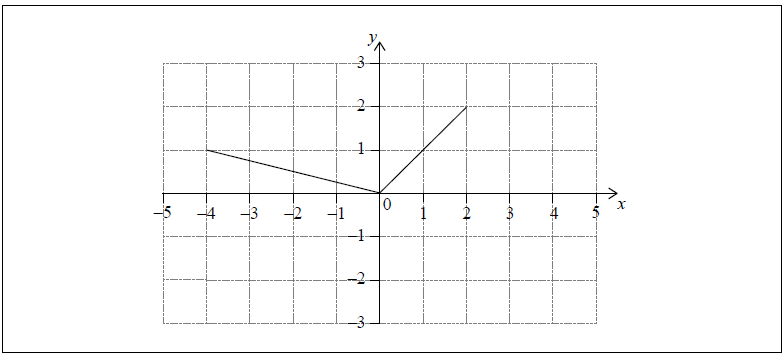On the same axes, sketch the graph of $$f\left( { – x} \right)$$.


a.

Another function, $$g$$, can be written in the form $$g\left( x \right) = a \times f\left( {x + b} \right)$$. The following diagram shows the graph of $$g$$.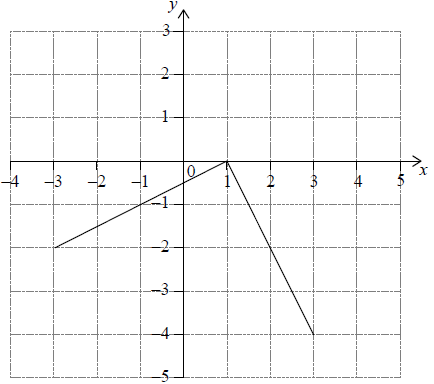Write down the value of a and of b.


b.

## Markscheme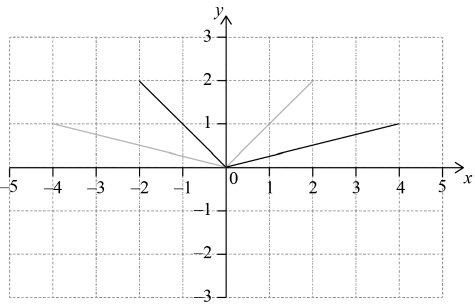A2 N2
[2 marks]

a.

recognizing horizontal shift/translation of 1 unit      (M1)

eg  = 1, moved 1 right

recognizing vertical stretch/dilation with scale factor 2      (M1)

eg   a = 2,  ×(−2)

a = −2,  b = −1     A1A1 N2N2

[4 marks]

b.

## Question

Consider a function $$f$$. The line L1 with equation $$y = 3x + 1$$ is a tangent to the graph of $$f$$ when $$x = 2$$

Let $$g\left( x \right) = f\left( {{x^2} + 1} \right)$$ and P be the point on the graph of $$g$$ where $$x = 1$$.

Write down $$f’\left( 2 \right)$$.


a.i.

Find $$f\left( 2 \right)$$.


a.ii.

Show that the graph of g has a gradient of 6 at P.


b.

Let L2 be the tangent to the graph of g at P. L1 intersects L2 at the point Q.

Find the y-coordinate of Q.


c.

## Markscheme

recognize that $$f’\left( x \right)$$ is the gradient of the tangent at $$x$$     (M1)

eg   $$f’\left( x \right) = m$$

$$f’\left( 2 \right) = 3$$  (accept m = 3)     A1 N2

[2 marks]

a.i.

recognize that $$f\left( 2 \right) = y\left( 2 \right)$$     (M1)

eg  $$f\left( 2 \right) = 3 \times 2 + 1$$

$$f\left( 2 \right) = 7$$     A1 N2

[2 marks]

a.ii.

recognize that the gradient of the graph of g is $$g’\left( x \right)$$      (M1)

choosing chain rule to find $$g’\left( x \right)$$      (M1)

eg  $$\frac{{{\text{d}}y}}{{{\text{d}}u}} \times \frac{{{\text{d}}u}}{{{\text{d}}x}},\,\,u = {x^2} + 1,\,\,u’ = 2x$$

$$g’\left( x \right) = f’\left( {{x^2} + 1} \right) \times 2x$$     A2

$$g’\left( 1 \right) = 3 \times 2$$     A1

$$g’\left( 1 \right) = 6$$     AG N0

[5 marks]

b.

at Q, L1L2 (seen anywhere)      (M1)

recognize that the gradient of L2 is g’(1)  (seen anywhere)     (M1)
eg  m = 6

finding g (1)  (seen anywhere)      (A1)
eg  $$g\left( 1 \right) = f\left( 2 \right),\,\,g\left( 1 \right) = 7$$

attempt to substitute gradient and/or coordinates into equation of a straight line      M1
eg  $$y – g\left( 1 \right) = 6\left( {x – 1} \right),\,\,y – 1 = g’\left( 1 \right)\left( {x – 7} \right),\,\,7 = 6\left( 1 \right) + {\text{b}}$$

correct equation for L2

eg  $$y – 7 = 6\left( {x – 1} \right),\,\,y = 6x + 1$$     A1

correct working to find Q       (A1)
eg   same y-intercept, $$3x = 0$$

$$y = 1$$     A1 N2

[7 marks]

c.

MAA SL 2.3-2.5 FUNCTIONS – COMPOSITION – INVERSE [concise]-lala

### Question

[Maximum mark: 6] [with / without GDC]
(a) On the same set of axes, sketch the graphs of the following two functions

f (x) = 2x ,             – 3 ≤ x ≤ 3
g(x) = 2x + 2 ,        – 3 ≤ x ≤ 3(b) Write down the range of each function (for the given domain).Ans.

In the domain – 3 ≤ x ≤ 3### Question

[Maximum mark: 8] [with GDC]
(a) On the same set of axes, sketch the graphs of the following two functions

f (x) = x2 ,             – 3 ≤ x ≤ 3
g(x) = x2 – 2 ,        – 3 ≤ x ≤ 3

For each function, write down
(b) the range (for the given domain);
(c) the intersection points with the line y = x .Ans.

In the domain – 3 ≤ x ≤ 3 (for the intersection points you may use the GDC)### Question

[Maximum mark: 5] [with GDC]
(a) Sketch the graph of the function $$f(x)=\sqrt{x},$$    $$0\leq x\leq 9$$.(b) Complete the following tableAns.

(a) Look at the graph in the GDC. Indicate the endpoints (0,0) and (9,3)(b) In the domain 0 ≤ x ≤ 9 (for the intersection points you may use the GDC)### Question

[Maximum mark: 6] [with GDC]
(a) Sketch the graph of the function $$f(x)= 3x-3\sqrt{x},$$     $$0\leq x\leq 4$$(b) Complete the following tableAns.

(a) Look at the graph in the GDC.Indicate the endpoints (0,0) and (4,6), the x-intercepts 0 and 1, and the minimum point.
(b)### Question

[Maximum mark: 10] [with GDC]
(a) Sketch the graphs of the following two functions. Write down the largest possible
domain and the corresponding range.(b) Write down the range of these functions under the following restrictionsAns.

(a) For $$f(x)=x^{3}$$          Df: x ∈ R                    Rf: y ∈ R

For $$f(x)=x^{4}$$                Df: x ∈ R                    Rf: y ∈ [0,+∞)

(b)### Question

[Maximum mark: 6] [without GDC]
Find the largest possible domain for the following functions

$$f(x)=3-6x$$              $$g(x)=\frac{7}{3-6x}$$                   $$h(x)=\sqrt{3-6x}$$                  $$k(x)= log(3-6x)$$

Ans.

Df: x ∈ R ,       Dg: x ≠ 1/ 2 ,       Dh: x ≤ 1/ 2 ,       Dk: x < 1/ 2 ,

### Question

[Maximum mark: 8] [with GDC]
Find the largest possible domain for the following functions

$$f(x)=x^{2}-4$$                 $$g(x)=\frac{7}{x^{2}-4}$$          $$h(x)=\sqrt{x^{2}-4}$$              $$k(x)=log({x^{2}-4})$$

Ans.

Df: x ∈ R ,       Dg: x≠±2 ,       Dh: x ≤ -2 or x ≥ 2,       Dk: x < -2 or x > 2

### Question

[Maximum mark: 6] [without GDC]
The function $$f$$ is given by $$f(x)=\sqrt{x-4}+\frac{2}{x-5}+log(10-x).$$

Find the largest possible domain of the function.

Ans.

Df: x ∈ [4,5) ∪ (5,10)    or     Df: 4 ≤ x < 5 or 5 < x < 10

### Question

[Maximum mark: 5] [with GDC]
The function $$f$$ is given by $$f(x)=\sqrt{x+7}+5$$

(a) Write down the domain of the function.
(b) Sketch the graph of the function (by using the GDC) to obtain the range of the
function

Ans.

Domain: x ≥ -7              Range: y ≥ 5

### Question

[Maximum mark: 8] [with GDC]
Let $$f(x)=2+cos(2x)-2sin(0.5x)$$ for $$0\leq x\leq 3,$$ where $$x$$ is in radians.

(a) On the grid below, sketch the curve of $$y = f (x)$$, indicating clearly the point P on the
curve where the function has a max or min value.(b) Write down the solutions of $$f (x) = 0$$.
(c) Write down the range of $$f$$.

Ans.

Note: no marks for work in degrees.(b) 1.26, 2.26
(c) -0.472 ≤ y < 3 (since the minimum point is (1.74,-0.472)

### Question

[Maximum mark: 6] [with GDC]
(a) On the following diagram, sketch the graphs of $$y=2^{x}$$ and $$y=cos\; x$$ for $$-2\leq x\leq 1$$(b) The equation $$2^{x}=cos\;x$$ has a solution between –2 and –1. Find this solution.
(c) Write down the x-intercept of the function $$g(x)=2^{x}-cos\;x$$, between –2 and –1.

Ans.

(a)(b) x = –1.29
(c) It is exactly the same x = –1.29

### Question

[Maximum mark: 6] [with GDC]
Let $$f(x)=sin(2x+1),0\leq x\leq π$$.

(a) Sketch the curve of $$y = f (x)$$ on the grid below.(b) Find the x-coordinates of the maximum and minimum points of $$f(x)$$, giving your
answers correct to one decimal place.

Ans.

(a)Note: graph crossing the y-axis between 0.5 and 1, curve crossing the x-axis twice.

(b) (Maximum) x = 0.285… $$\left ( \frac{\pi }{4}-\frac{1}{2} \right )x=0.3 (1\;d.p)$$

(Minimum) x = 1.856… $$\left ( \frac{3\pi }{4}-\frac{1}{2} \right )x=1.9 (1\;d.p)$$

### Question

[Maximum mark: 10] [with GDC]
Note: Radians are used throughout this question.
Let $$f (x) = sin (1 + sin x)$$.
(a) Sketch the graph of $$y = f (x)$$, for $$0 ≤ x ≤ 6$$.
(b) Write down the x-coordinates of all minimum and maximum points, for $$0 ≤ x ≤ 6$$.
(c) Write down the range of $$y = f (x)$$, for $$0 ≤ x ≤ 6$$.

Ans.

(a)(b) Maximum/minimum points at: 0.6075, 1.571, 2.534, 4.712
(c) [0,1]

### Question

[Maximum mark: 4] [with GDC]
The diagram below shows the graph of $$y=x\;sin\frac{x}{3}$$, for $$0 ≤ x < m$$, and $$0 ≤ y < n$$, where $$x$$
is in radians and $$m$$ and $$n$$ are integers.Find the value of $$m$$ and the value of $$n$$.

Ans.From a graphic display calculator
(a) $$y$$ = 0 ⇒ $$x$$ = 9.43 (or $$x$$ between 9 and 10)⇒ $$m$$ = 10
(b) $$y$$max = 5.46 (or between 5 and 6) ⇒ $$n$$ = 6

### Question

[Maximum mark: 6] [with GDC]
Let $$ƒ (x) = 3 sin 2x$$, for 1 ≤ x ≤ 4 and $$g(x)=-5x^{2}+27x-35$$ for 1 ≤ x ≤ 4. The graph of $$ƒ$$ is
shown below.(a) On the same diagram, sketch the graph of $$g$$.
(b) One solution of $$ƒ (x) = g (x)$$ is 1.89. Write down the other solution.
(c) Let $$h (x) = g (x) − ƒ (x)$$. Given that $$h (x) ≤ 0$$ for $$p ≤ x ≤ q$$, write down the value of $$p$$
and of $$q$$.

Ans.

(a)(b) 3.19
(c) $$p$$ = 1.89, $$q$$ = 3.19

## COMPOSITION OF FUNCTIONS – INVERSE FUNCTIONS

### Question

[Maximum mark: 10] [without GDC]
Let $$f (x) = 10-2x$$ and $$g(x) = 5x$$. Calculate

(a) (f◦g)(x) and (g◦f)(x)
(b) f -1(x)
(c) g -1(10)
(d) (f -1◦g)(x) and (g◦f ) -1(x)
(e) (f◦f )(x) and (g◦g)(x)

Ans.

(a) (f◦g)(x) = 10-10x            (g◦f)(x) = 50 – 10x
(b) f -1(x) = (10-x)/2,
(c) g -1(10) = 2
(d) (f -1 ◦g)(x) = (10-5x)/2, (g◦f ) -1(x) = (50-x)/10
(e) (f◦f)(x) = 4x-10 (g◦g)(x) = 25x

### Question

[Maximum mark: 8] [without GDC]
The tables below show some values of two functions $$f$$ and $$g$$(a) Write down the values of g(3), f -1(3)
(b) Calculate (f◦g)(2)
(c) Calculate (g◦g)(3)
(d) Find a solution of the equation (g◦f )(x) = 3

Ans.

(a) g(3) =1               f -1(3) =4
(b) (f◦g)(2) = -1
(c) (g◦g)(3) = 5
(d) x = 1

### Question

[Maximum mark: 5] [without GDC]
Find the inverse of the function $$f(x)=\frac{x}{x+5}$$

Ans.

$$f^{-1}(x)=\frac{-5x}{x-1}$$

### Question

[Maximum mark: 8] [without GDC]
Let $$f(x)=\frac{2x-3}{3x-2}$$. Show that

(a) f -1=f  (i.e. the function is self-inverse)

(b) ( f ◦ f )(x) = x

Ans.

Just confirm that $$f^{-1}(x)=\frac{2x-3}{3x-2}$$ and ( f ◦ f )(x) = x

### Question

[Maximum mark: 10] [without GDC]
The function $$f$$ is given by $$f(x)=x^{2}$$, for $$0\leq x\leq 3$$.

(a) Sketch the graph of $$f$$.
(b) State the domain and the range of $$f$$.
(c) Find the inverse function f –1.
(d) On the same axes with $$f$$, sketch the graph of f –1
(e) State the domain and the range of f –1.

Ans.

(a) parabola between $$x$$= 0 and $$x$$ = 3
(b) 0 ≤ x ≤ 3           0 ≤ y ≤ 9
(c) $$y=\sqrt{x}$$

(d)(e) 0 ≤ x ≤ 9          0 ≤ y ≤ 3

### Question

[Maximum mark: 6] [without GDC]
Let $$g(x)=3x-2$$,                  $$h(x)=\frac{5x}{x-4}$$,      $$x\neq 4$$.

(a) Find an expression for (h ◦ g) (x). Simplify your answer.
(b) Solve the equation (h ◦ g) (x) = 0.

Ans.

(a) (h ◦ g) (x) = $$\frac{5(3x-2)}{(3x-2)-4}=\frac{15x-10}{3x-6}$$

(b) numerator = $$0\Rightarrow M1)x=\frac{2}{3} (=0.667)$$

### Question

[Maximum mark: 6] [without GDC]
The functions $$f$$ and $$g$$ are defined by $$f:\mapsto 3x$$, $$g:x\mapsto x+2$$.

(a) Find an expression for (f ◦ g) (x).
(b) Show that f –1(18) + g–1(18) = 22.

Ans.

(a) (f ◦ g): $$x \mapsto 3(x+2)(=3x+6)$$

(b) METHOD 1

$$f^{-1}(x)=\frac{x}{3}$$                $$g^{-1}(x)=x-2$$

$$f^{-1}(18)=\frac{18}{3}=6$$              $$g^{-1}(18)=18-2=16$$

$$f^{-1}(18)+g^{-1}(18)=6+16=22$$

METHOD 2

3x = 18, x + 2 = 18
x = 6, x = 16
f –1(18) + g–1(18) = 6 +16 = 22

### Question

[Maximum mark: 6] [without GDC]
Let $$f(x)=2x+1$$ and $$g(x)=3x^{2}-4$$. Find

(a) f –1(x);
(b) (g ◦ f ) (–2);
(c) ( f ◦ g) (x).

Ans.

(a) $$y=2x+1 \Rightarrow x=\frac{y-1}{2}$$
$$f^{-1}(x)=\frac{x-1}{2}$$

(b) $$g(f(-2))=g(-3)=3(-3)^{2}-4=23$$

(c) $$f(g(x))=f(3x^{2}-4)=2(3x^{2}-4)+1=6x^{2}-7$$

### Question

[Maximum mark: 4] [without GDC]
The function $$f$$ is defined by $$f:x \mapsto \sqrt{3-2x}, x\leq \frac{3}{2}$$

Evaluate f –1(5).

Ans.

$$\sqrt{3-2x}=5\Leftrightarrow 3-2x=25\Leftrightarrow -2x=22\Leftrightarrow x=-11$$

OR

Let $$y=\sqrt{3-2x}\Rightarrow y^{2}=3-2x\Rightarrow x=\frac{3-y^{2}}{2}$$
$$\Rightarrow f^{-1}(x)=\frac{3-x^{2}}{2}\Rightarrow f^{-1}(5)=\frac{3-25}{2}=-11$$

### Question

[Maximum mark: 6] [without GDC]
Let $$f(x)=\sqrt{x+4} x\geq -4$$ and $$g(x)=x^{2}$$,  $$x \epsilon \mathbb{R}$$.
(a) Find (g ◦ f ) (3)
(b) Find f –1(x)
(c) Write down the domain of f –1 .

Ans.

(a) METHOD 1

$$f(3)=\sqrt{7}$$        (g ◦ f ) (3) = 7

METHOD 2

(g ◦ f) (x) = $$\sqrt{x+4}^{2}=x+4$$

(g ◦ f) (3) = 7

(b) $$y=\sqrt{x+4}\Rightarrow y^{2}=x+4\Rightarrow x=y^{2}-4$$
$$f^{-1}(x)=x^{2}-4$$

(c) x ≥ 0

### Question

[Maximum mark: 5] [without GDC]
Let $$f(x)=x^{3}-4$$ and $$g(x)=2x$$.

(a) Find (g ◦ f ) (−2).
(b) Find f −1 (x).

Ans.

(a) METHOD 1

f (-2) = – 12
(g ◦ f) (-2) = g (-12) = – 24

METHOD 2

(g ◦ f) (x) = 2x3 – 8
(g ◦ f) (-2) = – 24

(b) $$y=x^{3}-4\Rightarrow y+4=x^{3}\Rightarrow x=\sqrt{y+4}$$
$$f^{-1}(x)=\sqrt{(x+4)}$$

### Question

[Maximum mark: 6] [without GDC]
Consider the functions $$f(x)=2x$$ and $$g(x)=\frac{1}{x-3}$$,  $$x\neq 3$$.

(a) Calculate (f ◦ g )(4) .
(b) Find g-1(x)
(c) Write down the domain of g-1 .

Ans.

(a) METHOD 1
(f ◦ g) (4) = f (g (4)) = f (1) = 2

METHOD 2

(f ◦ g) (x) = $$\frac{3}{x-3}$$ so (f ◦ g) (4) = 2

(b) Let $$y=\frac{1}{x-3}\Rightarrow y(x-3)=1\Rightarrow x-3=\frac{1}{y}\Rightarrow x=\frac{1}{y}+3\left ( =\frac{1+3y}{y} \right )$$
$$g^{-1}(x)=\frac{1}{x}+3\left ( =\frac{1+3x}{x} \right )$$

(c) $$x\neq 0 (or \mathbb{R}$$ \ {0} etc)

### Question

[Maximum mark: 4] [without GDC]
Two functions $$f, g$$ are defined as follows: $$f:x \mapsto 3x+5$$ and $$g:x \mapsto 2(1-x)$$. Find

(a) f –1(2);
(b) (g ◦ f )(–4).

Ans.

(a) For $$f^{-1}(2), 3x+5=2\Rightarrow x=-1$$
$$f^{-1}(2)=-1$$

(b) g(f (–4) = g(–12 + 5) = g(–7) = 2(1 + 7) = 16

### Question

[Maximum mark: 6] [with GDC]
Two functions $$f$$ and $$g$$ are defined as follows: $$f (x) = cos\; x$$, $$g (x) = 2x + 1$$
(a) Find (g ◦ f) (x) and (f ◦ g) (x)
(b) Solve the equation (g ◦ f )(x) = 0 for 0 ≤ x ≤ 2π; [by using your GDC]

Ans.

(a) (g ° f ) (x) =2 cos x + 1 and (f ° g) (x) =2cos(2x + 1)
(b) By GDC  x = 2.09, 4.19 (in fact $$x=\frac{2\pi }{3},\frac{4\pi }{3}$$)

### Question

[Maximum mark: 6] [without GDC]
Consider the functions $$f:x \mapsto 4(x-1)$$ and $$g: x \mapsto \frac{6-x}{2}$$.

(a) Find g–1.
(b) Solve the equation ( f ◦ g–1) (x) = 4.

Ans.

(a) $$y=\frac{6-x}{2}\Rightarrow x=6-2y\Rightarrow g^{-1}(x)=6-2x$$

(b) (f ° g–1) (x) = $$4[(6-2x)-1]=4(5-2x)=20-8x$$
$$20-8x=4\Leftrightarrow 8x=16\Leftrightarrow x=2$$

### Question

[Maximum mark: 5] [without GDC]
Let $$f(x)=x^{2}$$ and $$g(x)=2x-3$$.

(a) Find g–1(x).
(b) Find (f ◦ g)(4).

Ans.

(a) $$y=2x-3\Rightarrow 2x=y+3\Rightarrow x=\frac{y+3}{2}$$      $$g^{-1}(x)=\frac{x+3}{2}$$

(b) METHOD 1
g(4) = 5 ⇒ f(5) = 25

METHOD 2
f ◦ g(x) = (2x – 3)2
f ◦ g(4) = (2 × 4 – 3)2 = 25

### Question

[Maximum mark: 5] [without GDC]
Let $$f(x)=7-2x$$ and $$g(x)=x+3$$

(a) Find (g ◦ f)(x).
(b) Write down g–1(x).
(c) Find (f ◦ g–1)(5).

Ans.

(a) (g ◦ f)(x) = 7 – 2x + 3 = 10 – 2x
(b) g–1(x) = x – 3
(c) METHOD 1
g–1(5) = 2
f (2) = 3
METHOD 2
(f ◦ g–1)(x) = 7 – 2(x – 3) = 13 – 2x
(f ◦ g–1)(5) = 3

### Question

[Maximum mark: 5] [without GDC]
Let $$f(x)=3x$$, $$g(x)=2x-5$$ and $$h(x)= (f ◦ g)(x)$$.

(a) Find h(x).
(b) Find h–1(x).

Ans.

(a) $$h(x) = f(2x – 5) = 6x – 15$$

(b) $$6x-15=y\Rightarrow 6x=y+15\Rightarrow x=\frac{y+15}{6}$$

$$h^{-1}(x)=\frac{x+15}{6}$$

### Question

[Maximum mark: 6] [with GDC]
Let $$f(x)=\sqrt{x}$$ and $$g(x)=2^{x}$$.

(a) Find (f –1 ◦ g)(x)
(b) Solve the equation (f –1◦ g)(x) = 16

Ans.

(a) f –1(x) = x2
(f –1 ◦ g)(x) = f –1(2x) = 22x

(b) 22x = 16 ⇒ 2x = 4 ⇒ x = 2

### Question

[Maximum mark: 6] [without GDC]
Let $$f(x)= 2^{x}$$ and $$g(x)=\frac{x}{x-2}$$,$$(x\neq 2)$$. Find

(a) (g ◦ f ) (3);

(b) g–1 (5).

Ans.

(a) f (3) = 23
(g ◦ f ) (3) = $$\frac{2^{3}}{2^{3}-2}=\frac{8}{6}=\frac{4}{3}$$

(b) $$\frac{x}{x-2}=y\Rightarrow y(x-2)=x\Rightarrow yx-2y=x\Rightarrow x(y-1)=2y\Rightarrow x=\frac{2y}{y-1}$$
$$g^{-1}(x)=\frac{2x}{x-1}$$
$$g^{-1}(5)=\frac{10}{(5-1)}=2.5$$

### Question

[Maximum mark: 6] [without GDC]
The function $$f$$ is defined by $$f:x \mapsto x^3$$.

Find an expression for g(x) in terms of x in each of the following cases

(a) (f ◦ g )(x) = x + 1;
(b) (g ◦ f )(x) = x + 1.

Ans.

(a) $$f(g(x))=x+1\Rightarrow [g(x)]^{3}=x+1$$
so $$g(x)=\sqrt{x+1}$$

(b) $$g(f(x))=x+1\Rightarrow g(x^{3})=x+1$$
so $$g(x)=\sqrt{x+1}$$

### Question

[Maximum mark: 6] [without GDC]
Consider the functions $$f$$ and $$g$$ where $$f(x)=3x-5$$ and $$g(x)=x-2$$.

Solve the equation (f −1 ◦ g) (x) = (g–1 ◦ f) (x).

Ans.

$$f^{-1}(x)=\frac{x+5}{3}$$             $$g^{-1}(x)=x+2$$

(f-1◦ g) (x) = $$\frac{x+3}{3}$$        (g–1 ◦ f) (x) = 3x – 3 ⇔

$$\frac{x+3}{3}=3x-3 \Leftrightarrow x+3=9x-9\Leftrightarrow x=\frac{12}{8}=\frac{3}{2}$$

### Question

[Maximum mark: 9] [with / without GDC]
The function $$f$$ is given by $$f(x)=x^{2}-6x+13$$, for $$x\geq 3$$.
(a) Show that $$f$$ may also be written in the form $$f(x)=(x-3)^{2}+4$$.
(b) Hence find the inverse function f –1.
(c) State the domain and the range of $$f$$ .
(d) State the domain and the range of f–1.

Ans.

(a) $$f(x)=(x-3)^{2}+4=x^{2}-6x+9+4=x^{2}-6x+13$$

(b) $$y=(x-3)^{2}+4$$
$$y-4=(x-3)^{2}$$
$$\sqrt{y-4}=x-3$$
$$\sqrt{y-4}+3=x$$
$$\Rightarrow f^{-1}(x)=\sqrt{x-4}+3$$

(c) x ≥ 3 and y ≥ 4
(d) x ≥ 4 and y ≥ 3

### Question

[Maximum mark: 7] [with GDC]
Consider the function $$f(x)=px^{3}+qx^{2}+rx$$. Part of the graph is shown below.The graph passes through the origin O and the points A(–2, –8), B(1, –2) and C(2, 0).
(a) Find three linear equations in $$p, q$$ and $$r$$.
(b) Hence find the value of $$p$$, of $$q$$ and of $$r$$.

(a) $$–8 = –8p + 4q – 2r$$
$$–2 = p + q + r$$
$$0 = 8p + 4q + 2r$$
(b) $$p$$ = 1, $$q$$ = –1, $$r$$ = –2